# Question:differentiating an integral w.r.t. a parameter

## Question:differentiating an integral w.r.t. a parameter

Maple

I woud like to have some help in using Maple to solve some complicated integrals.Specificaly, I would like to take the help of maple in differentiating an integral w.r.t. a parameter.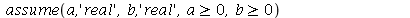Then, I will define my first function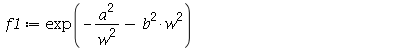If we integrate,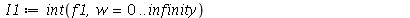we get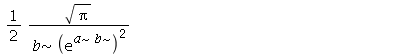Is there any way of completely removing the assumption sign for the parameters a and b? I don't need any reminders that I am asuming values (correct for my physical problem).

Now, I will define my next function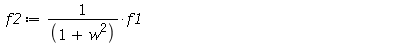Integrating this function, we get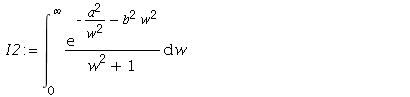Maple does not give a solution.

I have, on my own, shown that the solution is :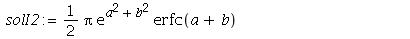I would like know how I can program Maple to get the same solution.

The first step in obtaining a soution is to differentiate the integral I2 with respect to b.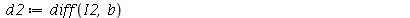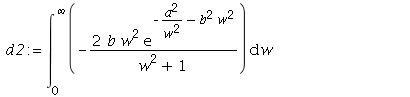I would ike to show that the solution ies in ing the differential equation (the integral I2 is denoted as I) :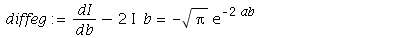I am new to Maple. Can I get some help here? Actually, I am interested in solving more complicated integrals. When I know how I can program in Maple, I can move on to these more complicated integrals.

When I first tried the integration w.r.t. the parameter b (with the assumptions), I could not get the solution shown above. Should I take away the assumptions (which is only logical when differentiang wrt it). Actually, I got the solution above only after I started formulating this question for this newsgroup. The reason why I started with the assumption is that I did not want any compex solutions (which I got for another problem). So, the question is really if it is possibe to relax the assumptions just for the sake of differentiating the integral.

On a side note, when verifying the solutions, I could not succeed in using evalf with pi. For example,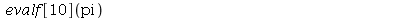does not give a numerical value.

Is there some way of forcing Maple to return a numerical value with a desired precision?

I will appreciate any help that I can get.

Thanks,

Ravi

﻿﻿